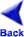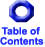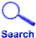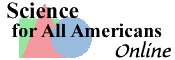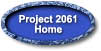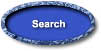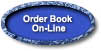## Chapter 9: THE MATHEMATICAL WORLD

### Chapter 9: THE MATHEMATICAL WORLD

Mathematics is essentially a process of thinking that involves building and applying abstract, logically connected networks of ideas. These ideas often arise from the need to solve problems in science, technology, and everyday life—problems ranging from how to model certain aspects of a complex scientific problem to how to balance a checkbook.

This chapter presents recommendations about basic mathematical ideas, especially those with practical application, that together play a key role in almost all human endeavors. In Chapter 2, mathematics is characterized as a modeling process in which abstractions are made and manipulated and the implications are checked out against the original situation. Here, the focus is on seven examples of the kinds of mathematical patterns that are available for such modeling: the nature and use of numbers, symbolic relationships, shapes, uncertainty, summarizing data, sampling data, and reasoning.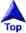### NUMBERS

There are several kinds of numbers that in combination with a logic for interrelating them form interesting abstract systems and can be useful in a variety of very different ways. The age-old concept of number probably originated in the need to count how many things there were in a collection of things. Thus, fingers, pebbles in containers, marks on clay tablets, notches on sticks, and knots on cords were all early ways of keeping track of and representing counted quantities. More recently, during the past 2,000 years or so, various systems of writing have been used to represent numbers. The Arabic number system, as commonly used today, is based on ten symbols (0, 1, 2, . . . 9) and rules for combining them in which position is crucial (for example, in 203, the 3 stands for three, the 2 stands for two hundreds, and the zero stands for no additional tens). In the binary system—the mathematical language of computers—just two symbols, 0 and 1, can be combined in a string to represent any number. The Roman number system, which is still used for some purposes (but rarely for calculation), is made up of a few letters of the alphabet and rules for combining them (for example, IV for four, X for ten, and XIV for fourteen, but no symbol for zero).

There are different kinds of numbers. The numbers that come from counting things are whole numbers, which are the numbers we mostly use in everyday life. A whole number by itself is an abstraction for how many things there are in a set but not for the things themselves. "Three" can refer to apples, boulders, people, or anything else. But in most practical situations, we want to know what the objects are, as well as how many there are. Thus, the answer to most calculations is a magnitude—a number connected to a label. If some people traveled 165 miles in 3 hours, their average speed was 55 miles per hour, not 55. In this instance, 165, 3, and 55 are numbers; 165 miles, 3 hours, and 55 miles per hour are magnitudes. The labels are important in keeping track of the meanings of the numbers.

Fractions are numbers we use to stand for a part of something or to compare two quantities. One common kind of comparison occurs when some magnitude such as length or weight is measured—that is, is compared to a standard unit such as a meter or a pound. Two kinds of symbols are widely used to stand for fractions, but they are numerically equivalent. For example, the ordinary fraction 3/4 and the decimal fraction 0.75 both represent the same number. Used to represent measured magnitudes, however, the two expressions may have somewhat different implications: 3/4 could be used to simply mean closer to 3/4 than to 2/4 or 4/4, whereas 0.75 may imply being closer to 0.75 than to 0.74 or 0.76—a much more precise specification. Whole numbers and fractions can be used together: 1 1/4, 1.25, 125/100, and 5/4, for instance, all mean the same thing numerically.

More flexibility in mathematics is provided by the use of negative numbers, which can be thought of in terms of a number line. A number line lays consecutive numbers at equal intervals along a straight line centered on zero. The numbers on one side of zero are called positive, and those on the other side, negative. If the numbers to the right of zero are positive, the numbers to the left of zero are negative; if distance above sea level is positive, distance below sea level is negative; if income is positive, debt is negative. If 2:15 is the scheduled time of lift-off, 2:10 is "minus 5 minutes." The complete range of numbers—positive, zero, and negative—allows any number to be subtracted from any other and still give an answer.

Computation is the manipulation of numbers and other symbols to arrive at some new mathematical statement. These other symbols may be letters used to stand for numbers. For example, in trying to solve a particular problem, we might let X stand for any number that would meet the conditions of the problem. There are also symbols to signify what operations to perform on the number symbols. The most common ones are +, -, x, and / (there are also others). The operations + and - are inverses of each other, just as x and / are: One operation undoes what the other does. The expression a/b can mean "the quantity a compared to the quantity b," or "the number you get if you divide a by b," or "a parts of size 1/b." The parentheses in a(b + c) tell us to multiply a by the sum of b and c. Mathematicians study systems of numbers to discover their properties and relationships and to devise rules for manipulating mathematical symbols in ways that give valid results.

Numbers have many different uses, some of which are not quantitative or strictly logical. In counting, for example, zero has a special meaning of nothing. Yet, on the common temperature scale, zero is only an arbitrary position and does not mean an absence of temperature (or of anything else). Numbers can be used to put things in an order and to indicate only which is higher or lower than others—not to specify by how much (for example, the order of winners in a race, street addresses, or scores on psychological tests for which numerical differences have no uniform meaning). And numbers are commonly used simply to identify things without any meaningful order, as in telephone numbers and as used on athletic shirts and license plates.

Aside from their application to the world of everyday experience, numbers themselves are interesting. Since earliest history, people have asked such questions as, Is there a largest number? A smallest number? Can every possible number be obtained by dividing some whole number by another? And some numbers, such as the ratio of a circle's circumference to its diameter (pi), catch the fancy of many people, not just mathematicians.### SYMBOLIC RELATIONSHIPS

Numbers and relationships among them can be represented in symbolic statements, which provide a way to model, investigate, and display real-world relationships. Seldom are we interested in only one quantity or category; rather, we are usually interested in the relationship between them—the relationship between age and height, temperature and time of day, political party and annual income, sex and occupation. Such relationships can be expressed by using pictures (typically charts and graphs), tables, algebraic equations, or words. Graphs are especially useful in examining the relationships between quantities.

Algebra is a field of mathematics that explores the relationships among different quantities by representing them as symbols and manipulating statements that relate the symbols. Sometimes a symbolic statement implies that only one value or set of values will make the statement true. For example, the statement 2A+4 = 10 is true if (and only if) A = 3. More generally, however, an algebraic statement allows a quantity to take on any of a range of values and implies for each what the corresponding value of another quantity is. For example, the statement A = s2 specifies a value for the variable A that corresponds to any choice of a value for the variable s.

There are many possible kinds of relationships between one variable and another. A basic set of simple examples includes (1) directly proportional (one quantity always keeps the same proportion to another), (2) inversely proportional (as one quantity increases, the other decreases proportionally), (3) accelerated (as one quantity increases uniformly, the other increases faster and faster), (4) converging (as one quantity increases without limit, the other approaches closer and closer to some limiting value), (5) cyclical (as one quantity increases, the other increases and decreases in repeating cycles), and (6) stepped (as one quantity changes smoothly, the other changes in jumps).

Symbolic statements can be manipulated by rules of mathematical logic to produce other statements of the same relationship, which may show some interesting aspect more clearly. For example, we could state symbolically the relationship between the width of a page, P, the length of a line of type, L, and the width of each vertical margin, m: P = L+2m. This equation is a useful model for determining page makeup. It can be rearranged logically to give other true statements of the same basic relationship: for example, the equations L = P-2m or m = (P-L)/2, which may be more convenient for computing actual values for L or m.

In some cases, we may want to find values that will satisfy two or more different relationships at the same time. For example, we could add to the page-makeup model another condition: that the length of the line of type must be 2/3 of the page width: L = 2/3P. Combining this equation with m = (P-L)/2, we arrive logically at the result that m = 1/6P. This new equation, derived from the other two together, specifies the only values for m that will fit both relationships. In this simple example, the specification for the margin width could be worked out readily without using the symbolic relationships. In other situations, however, the symbolic representation and manipulation are necessary to arrive at a solution—or to see whether a solution is even possible.

Often, the quantity that interests us most is how fast something is changing rather than the change itself. In some cases, the rate of change of one quantity depends on some other quantity (for example, change in the velocity of a moving object is proportional to the force applied to it). In some other cases, the rate of change is proportional to the quantity itself (for example, the number of new mice born into a population of mice depends on the number and gender of mice already there).### SHAPES

Spatial patterns can be represented by a fairly small collection of fundamental geometrical shapes and relationships that have corresponding symbolic representation. To make sense of the world, the human mind relies heavily on its perception of shapes and patterns. The artifacts around us (such as buildings, vehicles, toys, and pyramids) and the familiar forms we see in nature (such as animals, leaves, stones, flowers, and the moon and sun) can often be characterized in terms of geometric form. Some of the ideas and terms of geometry have become part of everyday language. Although real objects never perfectly match a geometric figure, they more or less approximate them, so that what is known about geometric figures and relationships can be applied to objects. For many purposes, it is sufficient to be familiar with points, lines, planes; triangles, rectangles, squares, circles, and ellipses; rectangular solids and spheres; relationships of similarity and congruence; relationships of convex, concave, intersecting, and tangent; angles between lines or planes; parallel and perpendicular relationships between lines and planes; forms of symmetry such as displacement, reflection, and rotation; and the Pythagorean theorem.

Both shape and scale can have important consequences for the performance of systems. For example, triangular connections maximize rigidity, smooth surfaces minimize turbulence, and a spherical container minimizes surface area for any given mass or volume. Changing the size of objects while keeping the same shape can have profound effects owing to the geometry of scaling: Area varies as the square of linear dimensions, and volume varies as the cube. On the other hand, some particularly interesting kinds of patterns known as fractals look very similar to one another when observed at any scale whatever—and some natural phenomena (such as the shapes of clouds, mountains, and coastlines) seem to be like that.

Geometrical relationships can also be expressed in symbols and numbers, and vice versa. Coordinate systems are a familiar means of relating numbers to geometry. For the simplest example, any number can be represented as a unique point on a line—if we first specify points to represent zero and one. On any flat surface, locations can be specified uniquely by a pair of numbers or coordinates. For example, the distance from the left side of a map and the distance from the bottom, or the distance and direction from the map's center.

Coordinate systems are essential to making accurate maps, but there are some subtleties. For example, the approximately spherical surface of the earth cannot be represented on a flat map without distortion. Over a few dozen miles, the problem is barely noticeable; but on the scale of hundreds or thousands of miles, distortion necessarily appears. A variety of approximate representations can be made, and each involves a somewhat different kind of distortion of shape, area, or distance. One common type of map exaggerates the apparent areas of regions close to the poles (for example, Greenland and Alaska), whereas other useful types misrepresent what the shortest distance between two places is, or even what is adjacent to what.

Mathematical treatment of shape also includes graphical depiction of numerical and symbolic relationships. Quantities are visualized as lengths or areas (as in bar and pie charts) or as distances from reference axes (as in line graphs or scatter plots). Graphical display makes it possible to readily identify patterns that might not otherwise be obvious: for example, relative sizes (as proportions or differences), rates of change (as slopes), abrupt discontinuities (as gaps or jumps), clustering (as distances between plotted points), and trends (as changing slopes or projections). The mathematics of geometric relations also aids in analyzing the design of complex structures (such as protein molecules or airplane wings) and logical networks (such as connections of brain cells or long-distance telephone systems).### UNCERTAINTY

Probability

Our knowledge of how the world works is limited by at least five kinds of uncertainty: (1) inadequate knowledge of all the factors that may influence something, (2) inadequate number of observations of those factors, (3) lack of precision in the observations, (4) lack of appropriate models to combine all the information meaningfully, and (5) inadequate ability to compute from the models. It is possible to predict some events with great accuracy (eclipses), others with fair accuracy (elections), and some with very little certainty (earthquakes). Although absolute certainty is often impossible to attain, we can often estimate the likelihood—whether large or small—that some things will happen and what the likely margin of error of the estimate will be.

It is often useful to express likelihood as a numerical probability. We usually use a probability scale of 0 to 1, where 0 indicates our belief that some particular event is certain not to occur, 1 indicates our belief that it is certain to occur, and anything in between indicates uncertainty. For example, a probability of .9 indicates a belief that there are 9 chances in 10 of an event occurring as predicted; a probability of .001 indicates a belief that there is only 1 chance in 1,000 of its occurring. Equivalently, probabilities can also be expressed as percentages, ranging from 0 percent (no chance) to 100 percent (certainty). Uncertainties can also be expressed as odds: A probability of .8 for an event can be expressed as odds of 8 to 2 (or 4 to 1) in favor of its occurring.

One way to estimate the probability of an event is to consider past events. If the current situation is similar to past situations, then we may expect somewhat similar results. For example, if it rained on 10 percent of summer days last year, we could expect that it will rain on approximately 10 percent of summer days this year. Thus, a reasonable estimate for the probability of rain on any given summer day is .1—one chance in ten. Additional information can change our estimate of the probability. For example, rain may have fallen on 40 percent of the cloudy days last summer; thus, if our given day is cloudy, we would raise the estimate from .1 to .4 for the probability of rain. The more ways in which the situation we are interested in is like those for which we have data, the better our estimate is likely to be.

Another approach to estimating probabilities is to consider the possible alternative outcomes to a particular event. For example, if there are 38 equally wide slots on a roulette wheel, we may expect the ball to fall in each slot about 1/38 of the time. Estimates of such a theoretical probability rest on the assumption that all of the possible outcomes are accounted for and all are equally likely to happen. But if that is not true—for example, if the slots are not of equal size or if sometimes the ball flies out of the wheel—the calculated probability will be wrong.

Probabilities are most useful in predicting proportions of results in large numbers of events. A flipped coin has a 50 percent chance of coming up heads, although a person will usually not get precisely 50 percent heads in an even number of flips. The more times one flips it, the less likely one is to get a count of precisely 50 percent but the closer the proportion of heads is likely to be to the theoretical 50 percent. Similarly, insurance companies can usually come within a percentage point or two of predicting the proportion of people aged 20 who will die in a given year but are likely to be off by thousands of total deaths—and they have no ability whatsoever to predict whether any particular 20-year-old will die. In other contexts, too, it is important to distinguish between the proportion and the actual count. When there is a very large number of similar events, even an outcome with a very small probability of occurring can occur fairly often. For example, a medical test with a probability of 99 percent of being correct may seem highly accurate—but if that test were performed on a million people, approximately 10,000 individuals would receive false results.Summarizing Data

Information is all around us—often in such great quantities that we are unable to make sense of it. A set of data can be represented by a few summary characteristics that may reveal or conceal important aspects of it. Statistics is a form of mathematics that develops useful ways for organizing and analyzing large amounts of data. To get an idea of what a set of data is like, for example, we can plot each case on a number line, and then inspect the plot to see where cases are piled up, where some are separate from the others, where the highest and lowest are, and so on. Alternatively, the data set can be characterized in a summary fashion by describing where its middle is and how much variation there is around that middle.

The most familiar statistic for summarizing a data distribution is the mean, or common average; but care must be taken in using or interpreting it. When data are discrete (such as number of children per family), the mean may not even be a possible value (for example, 2.2 children). When data are highly skewed toward one extreme, the mean may not even be close to a typical value. For example, a small fraction of people who have very large personal incomes can raise the mean considerably higher than the bulk of people piled at the lower end can lower it. The median, which divides the lower half of the data from the upper half, is more meaningful for many purposes. When there are only a few discrete values of a quantity, the most informative kind of average may be the mode, which is the most common single value—for example, the most common number of cars per U.S. family is 1.

More generally, averages by themselves neglect variation in the data and may imply more uniformity than exists. For example, the average temperature on the planet Mercury of about 15o F does not sound too bad—until one considers that it swings from 300o F above to almost 300o F below zero. The neglect of variation can be particularly misleading when averages are compared. For example, the fact that the average height of men is distinctly greater than that of women could be reported as "men are taller than women," whereas many women are taller than many men. To interpret averages, therefore, it is important to have information about the variation within groups, such as the total range of data or the range covered by the middle 50 percent. A plot of all the data along a number line makes it possible to see how the data are spread out.

We are often presented with summary data that purport to demonstrate a relationship between two variables but lack essential information. For example, the claim that "more than 50 percent of married couples who have different religions eventually get divorced" would not tell us anything about the relationship between religion and divorce unless we also knew the percentage of couples with the same religion who get divorced. Only the comparison of the two percentages could tell us whether there may be a real relationship. Even then, caution is necessary because of possible bias in how the samples were selected and because differences in percentage could occur just by chance in selecting the sample. Proper reports of such information should include a description of possible sources of bias and an estimate of the statistical uncertainty in the comparison.

Two quantities are positively correlated if having more of one is associated with having more of the other. (A negative correlation means that having more of one is associated with having less of the other.) But even a strong correlation between two quantities does not mean that one is necessarily a cause of the other. Either one could possibly cause the other, or both could be the common result of some third factor. For example, life expectancy in a community is positively correlated with the average number of telephones per household. One could look for an explanation for how having more telephones improves one's health or why healthier people buy more telephones. More likely, however, both health and number of telephones are the consequence of the community's general level of wealth, which affects the overall quality of nutrition and medical care, as well as the people's inclination to buy telephones.Sampling

Most of what we learn about the world is obtained from information based on samples of what we are studying—samples of, say, rock formations, light from stars, television viewers, cancer patients, whales, or numbers. Samples are used because it may be impossible, impractical, or too costly to examine all of something, and because a sample often is sufficient for most purposes.

In drawing conclusions about all of something from samples of it, two major concerns must be taken into account. First, we must be alert to possible bias created by how the sample was selected. Common sources of bias in drawing samples include convenience (for example, interviewing only one's friends or picking up only surface rocks), self-selection (for example, studying only people who volunteer or who return questionnaires), failure to include those who have dropped out along the way (for example, testing only students who stay in school or only patients who stick with a course of therapy), and deciding to use only the data that support our preconceptions.

A second major concern that determines the usefulness of a sample is its size. If sampling is done without bias in the method, then the larger the sample is, the more likely it is to represent the whole accurately. This is because the larger a sample is, the smaller the effects of purely random variations are likely to be on its summary characteristics. The chance of drawing a wrong conclusion shrinks as the sample size increases. For example, for samples chosen at random, finding that 600 out of a sample of 1,000 have a certain feature is much stronger evidence that a majority of the population from which it was drawn have that feature than finding that 6 out of a sample of 10 (or even 9 out of the 10) have it. On the other hand, the actual size of the total population from which a sample is drawn has little effect on the accuracy of sample results. A random sample of 1,000 would have about the same margin of error whether it were drawn from a population of 10,000 or from a similar population of 100 million.### REASONING

Some aspects of reasoning have clear logical rules, others have only guidelines, and still others have almost unlimited room for creativity (and, of course, error). A convincing argument requires both true statements and valid connections among them. Yet formal logic concerns the validity of the connections among statements, not whether the statements are actually true. It is logically correct to argue that if all birds can fly and penguins are birds, then penguins can fly. But the conclusion is not true unless the premises are true: Do all birds really fly, and are penguins really birds? Examination of the truth of premises is as important to good reasoning as the logic that operates on them is. In this case, because the logic is correct but the conclusion is false (penguins cannot fly), one or both of the premises must be false (not all birds can fly, and/or penguins are not birds).

Very complex logical arguments can be built from a small number of logical steps, which hang on precise use of the basic terms "if," "and," "or," and "not." For example, medical diagnosis involves branching chains of logic such as "If the patient has disease X or disease Y and also has laboratory result B, but does not have a history of C, then he or she should get treatment D." Such logical problem solving may require expert knowledge of many relationships, access to much data to feed into the relationships, and skill in deducing branching chains of logical operations. Because computers can store and retrieve large numbers of relationships and data and can rapidly perform long series of logical steps, they are being used increasingly to help experts solve complex problems that would otherwise be very difficult or impossible to solve. Not all logical problems, however, can be solved by computers.

Logical connections can easily be distorted. For example, the proposition that all birds can fly does not imply logically that all creatures that can fly are birds. As obvious as this simple example may seem, distortion often occurs, particularly in emotionally charged situations. For example: All guilty prisoners refuse to testify against themselves; prisoner Smith refuses to testify against himself; therefore, prisoner Smith is guilty.

Distortions in logic often result from not distinguishing between necessary conditions and sufficient conditions. A condition that is necessary for a consequence is always required but may not be enough in itself—being a U.S. citizen is necessary to be elected president, for example, but not sufficient. A condition that is sufficient for a consequence is enough by itself, but there may be other ways to arrive at the same consequence—winning the state lottery is sufficient for becoming rich, but there are other ways. A condition, however, may be both necessary and sufficient; for example, receiving a majority of the electoral vote is both necessary for becoming president and sufficient for doing so, because it is the only way.

Logic has limited usefulness in finding solutions to many problems. Outside of abstract models, we often cannot establish with confidence either the truth of the premises or the logical connections between them. Precise logic requires that we can make declarations such as "If X is true, then Y is true also" (a barking dog does not bite), and "X is true" (Spot barks). Typically, however, all we know is that "if X is true, then Y is often true also" (a barking dog usually does not bite) and "X seems to be approximately true a lot of the time" (Spot usually barks). Commonly, therefore, strict logic has to be replaced by probabilities or other kinds of reasoning that lead to much less certain results—for example, to the claim that on average, rain will fall before evening on 70 percent of days that have morning weather conditions similar to today's.

If we apply logical deduction to a general rule (all feathered creatures fly), we can produce a conclusion about a particular instance or class of instances (penguins fly). But where do the general rules come from? Often they are generalizations made from observations—finding a number of similar instances and guessing that what is true of them is true of all their class ("every feathered creature I have seen can fly, so perhaps all can"). Or a general rule may spring from the imagination, by no traceable means, with the hope that some observable aspects of phenomena can be shown to follow logically from it (example: "What if it were true that the sun is the center of motion for all the planets, including the earth? Could such a system produce the apparent motions in the sky?").

Once a general rule has been hypothesized, by whatever means, logic serves in checking its validity. If a contrary instance is found (a feathered creature that cannot fly), the hypothesis is not true. On the other hand, the only way to prove logically that a general hypothesis about a class is true is to examine all possible instances (all birds), which is difficult in practice and sometimes impossible even in principle. So it is usually much easier to prove general hypotheses to be logically false than to prove them to be true. Computers now sometimes make it possible to demonstrate the truth of questionable mathematical generalizations convincingly, even if not to prove them, by testing enormous numbers of particular cases.

Science can use deductive logic if general principles about phenomena have been hypothesized, but such logic cannot lead to those general principles. Scientific principles are usually arrived at by generalizing from a limited number of experiences—for instance, if all observed feathered creatures hatch from eggs, then perhaps all feathered creatures do. This is a very important kind of reasoning even if the number of observations is small (for example, being burned once by fire may be enough to make someone wary of fire for life). However, our natural tendency to generalize can also lead us astray. Getting sick the day after breaking a mirror may be enough to make someone afraid of broken mirrors for life. On a more sophisticated level, finding that several patients having the same symptoms recover after using a new drug may lead a doctor to generalize that all similar patients will recover by using it, even though recovery might have occurred just by chance.

The human tendency to generalize has some subtle aspects. Once formed, generalities tend to influence people's perception and interpretation of events. Having made the generalization that the drug will help all patients having certain symptoms, for example, the doctor may be likely to interpret a patient's condition as having improved after taking the drug, even if that is doubtful. To prevent such biases in research, scientists commonly use a "blind" procedure in which the person observing or interpreting results is not the same person who controls the conditions (for instance, the doctor who judges the patient's condition does not know what specific treatment that patient received).

Much of reasoning, and perhaps most of creative thought, involves not only logic but analogies. When one situation seems to resemble another in some way, we may believe that it resembles it in other ways too. For example, light spreads away from a source much as water waves spread from a disturbance, so perhaps light acts like water waves in other ways, such as producing interference patterns where waves cross (it does). Or, the sun is like a fire in that it produces heat and light, so perhaps it too involves burning fuel (in fact, it does not). The important point is that reasoning by analogy can suggest conclusions, but it can never prove them to be true.